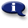# .

## Syllabus Information

Fall 2022
Mar 31,2023Use this page to maintain syllabus information, learning objectives, required materials, and technical requirements for the course.

Syllabus Information
MTH 112 - Trigonometry
Associated Term: Fall 2022
Learning Objectives: Upon successful completion of this course, the student will be able to:
1. Use geometry, algebra, and graphing calculator skills from previous courses
2. Move easily between degree and radian measure
3. Identify and use the six trigonometric functions in right triangle applications
4. Identify features of and use of the six circular functions
5. Graph the six circular functions and related transformations and extract features from their equations and graphs
6. Recall and apply the basic trigonometric identities
7. Use the sum, difference, double-angle, and half-angle identities
8. Identify features of and use the three major inverse trigonometric functions
9. Solve trigonometric equations analytically and with graphing technology
10. Apply the Law of Sines and Law of Cosines where appropriate
11. Use polar coordinates and polar equations and transform them to rectangular form and back
12. Use complex numbers in standard form and in polar form (time permitting)
13. Solve problems using vector notation
14. Use parametric equations
15. Work with the definitions, equations, and graphs of conic sections
16. Apply geometric and trigonometric relationships to applied problems
17. Use a graphing calculator to graph equations and explore concepts for equations in rectangular, parametric, or polar form
Required Materials:
Technical Requirements: